# Creating a Complex Skip Logic Expression

Complex Skip Logic (CSL) allows you to show or hide a question based on the answer(s) to one or more previously answered questions.

This video explains how to construct a Complex Skip Logic expression:

How to Create a Complex Skip Logic (CSL) Expression

A CSL Expression follows the following format:

if(Condition;What to do if True;What to do if False)

It looks like the following when filled out:

if(e1~=3;e4;e5)

Condition

The Condition is a logical test which can be evaluated as either True or False.

The Condition takes the format of the letter e followed directly by the Question ID for the question which is having its answer checked. This is followed by a logical test. And this is followed by the answer number being checked by the test.

Examples:

e2==3 tests if the response for Question 2 is Answer 3 and only Answer 3.

e5~=2 tests if the response for Question 5 contains Answer 2.

Skipping to End Screen

If you are writing a CSL statement where you will be skipping to the End Screen, use "end" in your CSL statement.

Examples:

if(e1==1;e2;end)

if(e7~=3;end;e12)

Logical Tests

There are two tests you can apply in the Condition part of the CSL Expression

Is Exactly (==)

You may evaluate if the response to a question is exactly an answer. The symbol used to represent this is ==. If you use the == symbol you are testing to see if a response is exactly an answer, and contains no more than that answer.

Example:If the condition you test for is e1==3, you are testing to see if the survey-taker has selected only Answer 3: Vegetables, and none of the other answers.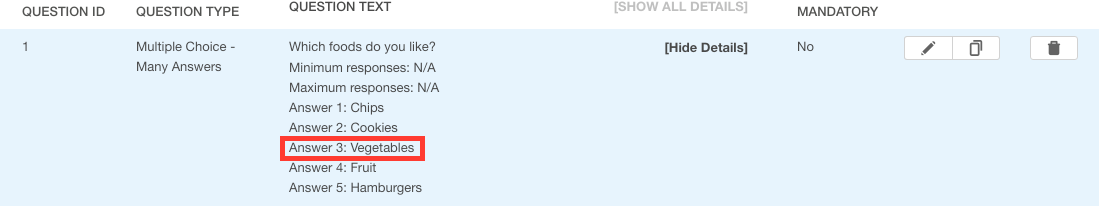If they respond to Question 1 with Answer 2: CookiesAnswer 3: Vegetables, and Answer 5: Hamburgerse1==3 will evaluate asFalse as more than only Answer 3: Vegetables, was selected.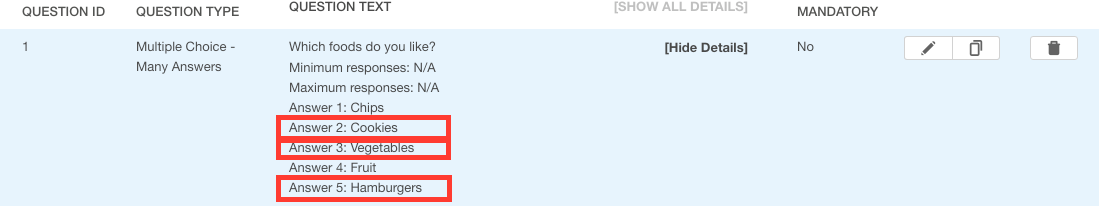If they respond to Question 1 with only Answer 3: Vegetablese1==3 will evaluate as True as only Answer 3: Vegetables, was selected.Example of an Is Exactly Condition in a CSL Expression:

if(e1==3;What to do if True;What to do if False)

Contains (~=)

You may evaluate if the response to a question contains an answer. The symbol used to represent this is ~=. If you use the ~= symbol you are testing to see if a response contains an answer, even if other responses are also selected.

Example: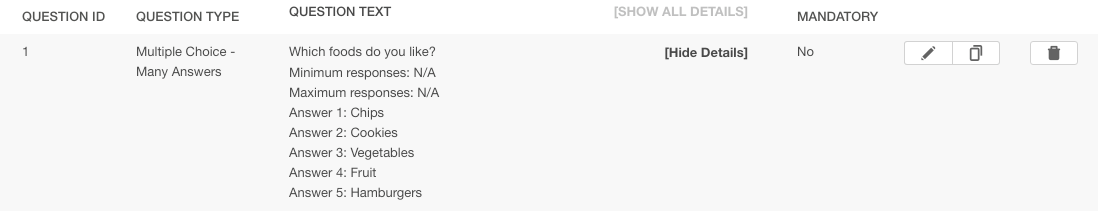If the condition you test for is e1~=3 you are testing to see if the survey-taker has selected Answer 3: Vegetables, even if they have also selected other answers.If they respond to Question 1 with only Answer 3: Vegetablese1~=3 will still evaluate as True as their response Answer 3: Vegetables, is contained in their response.Example of a Contains Condition in a CSL Expression:

if(e1~=3;What to do if True;What to do if False)

What to do if True

This part of the CSL Expression is evaluated if the condition is evaluated as being True. There are two options regarding what can be placed in the What to do if True part of the CSL Expression.

Option 1 - Tell the QuickTapSurvey App to Display a Question

You may tell the QuickTapSurvey App to present a specific question to the survey-taker by providing the Question ID in the form of efollowed directly by the Question ID. (Example: Question 4 would be represented by e4)

Example:

You want to tell the QuickTapSurvey App to display Question 4 if the condition is True it would look like the following.

if(e1~=3;e4;What to do if False)

Option 2 - Tell the QuickTapSurvey App to Evaluate Another Condition

You may place a whole other CSL Expression inside of the True part of the CSL Expression. This is called a nested CSL Expression.

Example:

You want to tell the QuickTapSurvey App to evaluate another condition if the condition is True it would look like the following.

if(e1~=3;if(e2~=3;e4;What to do if False);What to do if False)

What to do if False

This part of the CSL Expression is evaluated if the condition is evaluated as being False. There are two options regarding what can be placed in the What to do if False part of the CSL Expression.

Option 1 - Tell the QuickTapSurvey App to Display a Question

You may tell the QuickTapSurvey App to go to present a specific question to the survey-taker by providing the Question ID in the form of efollowed directly by the Question ID. (Example: Question 5 would be represented by e5)

Example:

You want to tell the QuickTapSurvey App to display Question 5 if the condition is False it would look like the following.

if(e1~=3;What to do if True;e5)

Option 2 - Tell the QuickTapSurvey App to Evaluate Another Condition

You may place a whole other CSL Expression inside of the False part of the CSL Expression. This is called a nested CSL Expression.

Example:

You want to tell the QuickTapSurvey App to evaluate another condition if the condition is False it would look like the following.

if(e1~=3;What to do if True;if(e2~=3;What to do if True;e5))

How to Create a Complex Skip Logic Expression

This how to section will show you how to create a CSL Expression by following the example scenario of a school which would like to ask certain students “Have you applied to college?” but only if they are in their Senior Year and also have a GPA of 3.0 or greater.

1. Ensure your survey has at least three questions. We suggest adding all questions to a survey before adding skip logic as moving questions after adding skip logic may impact the flow of the survey.

2. Decide which question you would like to skip or display based on a CSL Expression. In this example we are using Question 3.3. Decide which question(s) you would like to base the Condition for your CSL Expression upon. In this example the CSL Expression will contain conditions testing the responses to Question 1 and Question 2.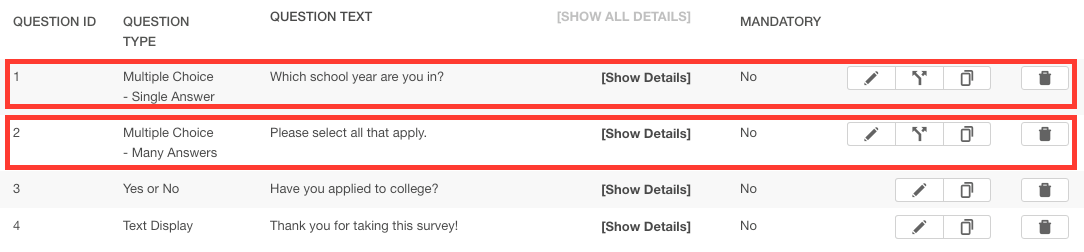4. Click Show Details for the questions which your CSL Expression will be based upon. In this example we will Show Details for Question 1and Question 2.5. Make a note of the answers which you will be testing in the CSL Expression. In this case we note that we only want Question 3 to be displayed if the response to Question 1 is exactly Answer 4 and the response to Question 2 contains Answer 5.6. Make a note regarding what you would like the survey to do if the CSL Expression is evaluated as False. In this example we note that if the CSL Expression is evaluated as False we would like the survey to display Question 4.7. Click the Edit Question icon for the question for which you would like to add a CSL Expression. In this case we will click on the Edit Question icon for Question 3.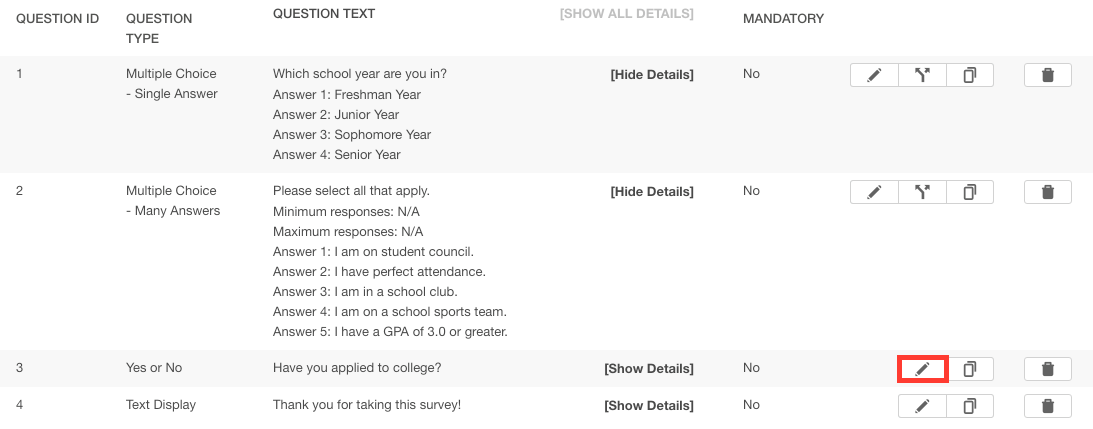8. Construct your CSL Expression based on your notes. In this example we will construct the CSL Expressionif(e1==4;if(e2~=5;e3;e4);e4)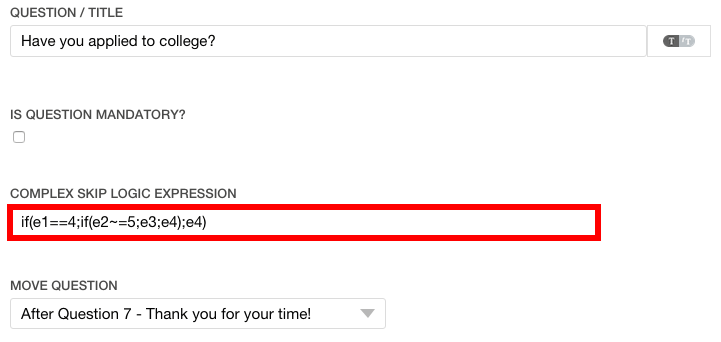9. Click the Save button.Note: CSL Expressions cannot tell the QuickTapSurvey App to skip backwards to a previous question.